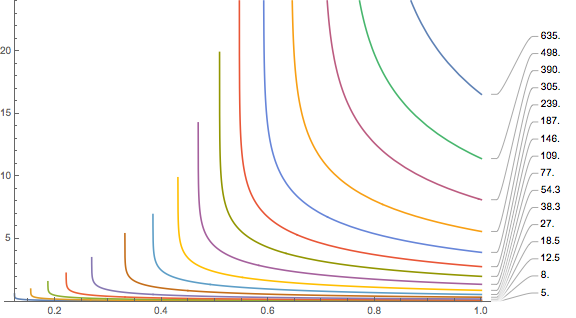## Can we calculate no-fly-times?

There are various recommendations how long one should not fly after surfacing from a dive. DAN has recently done a study where they did Doppler measurements on a plane and recommends an interval of 12 hours after a single dive and 24 hours after repetitive diving.

But one would think that it should be possible to use a decompression model that works well under water to compute such a time. So let’s do this in this post or at least compute a conservative estimate. To be specific, we will use the Buhlmann model. We do that calculation compartment by compartment and assume that when leaving the water, the tissue has the maximally allowed partial pressure for surfacing (i.e. this tissue was the guiding tissue for the final part of the ascent). Clearly, this is a conservative assumption. Then, according to the model

$$p_s = (p_i -a)b.$$

Here, ps is the surface pressure and a and b are the usual Bühlmann coefficients.

Then we do a surface interval (whose length we wish to determine in the end) during which the partial pressure decays exponentially:

$$p_i(t) = f_{N_2} p_s+ (p_i(0) – f_{N_2}p_s)e^{-\gamma t}$$

where f is the N2 fraction the the breathing gas (0.79 for air which we are probably breathing while waiting for the plane to board). Finally, we don’t want the cabin pressure to be less than the minimal ambient pressure that Dr. Bühlmann recommends. We want to parametrize the cabin pressure using the barometric formula which asserts (assuming constant temperature) that the pressure drops exponentially at height with decay constant that the pressure is 1/e at about hs=8500m above sea level. So, we set

$$e^{-h/hs} p_s= (p_i(t) -a)b.$$

This we can then solve for t. Actually, being conservative, we want to throw in gradient factors. Again, being conservative, we don’t further linearly extrapolate gradient factors, but will use GFhi everywhere on the surface. Plugging everything in (with the help of Mathematica and some manual massaging) we find

$$e^{\gamma t} = \frac{a GF/p_s + 1-f_{N_2} + GF(1/b -1)}{a GF/p_s – f_{N_2} +e^{-h/h_s}(1+GF(1/b-1))}.$$

Now, we have to plug in numbers. For the cabin pressure, according to Wikipedia, we will assume h=2400m for older aircrafts. The wait times (in hours) for the different compartments are then shown in this plot:

You are supposed to see a number of things:

• The waiting time depends strongly on which tissue we are dealing with. For reasonably large gradient factors, only the tissues with half-times of several hours contribute significant waiting times. Remember, for this calculation, we assumed the loading is at its maximal value when you get out of the water. For realistic sports/tec-diving scenarios (as opposed to saturation diving) that should be quite hard even on a weeklong liveaboard  with at least five dives a day. If slightly faster tissues are leading, the inferred no-fly times are much shorter, probably shorter than the queue at check-in. I looked at some data from real dive trips where people got everything out of their booked liveaboard but they got nowhere close to exciting the slow tissues. In the Subsurface planner I had to do five consecutive 2-hour dives with less than two hour surface interval to see at least some ceiling for the 239 minute compartment. In the plots, this has the blue line and leads to a no-fly time of much less than 5 hours.
• The plot ends on the left (small gradient factors) with diverging values. These divergences move to higher gradient factors when you increase the cabin pressurisation equivalent altitude (for example by assuming a loss of cabin pressure, remember this is when the oxygen masks are supposed to drop from the ceiling)Same plot with 4500m of altitude

This comes about as the no-fly time becomes infinite or even complex as according to the Bühlmann-with-gradient-factors limit, your are not allowed to be at the ambient pressure at that altitude even when saturated with the nitrogen that you experience at sea level. We can compute this limiting altitude by solving
$$f_{N_2} p_s = e^{-h/h_s} p_s (GF/b-GF +1) + a GF /p_s$$
for the altitude via
$$e^{-h/h_s} = \frac{f- aGF/p_s}{GF/b -GF +1}.$$
This is shown here, the maximum altitude after waiting an infinite amount of time: